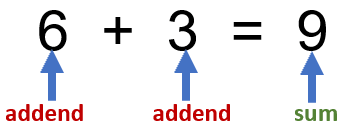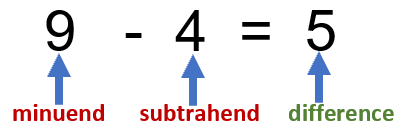# Math Worksheets Land

Math Worksheets For All Ages

# Math Worksheets Land

Math Worksheets For All Ages

# Adding and Subtracting Within 1000 Worksheets

### Aligned Standard: Grade 2 Base Ten - 2.NBT.7

• Step-by-step Lesson- Work on sums and differences of three digit numbers. This lesson provides six examples.
• Guided Lesson - Focused on three digit numbers A) Add horizontal B) Subtract vertically C) Add visually.
• Guided Lesson Explanation - I touch on the concept of carrying numbers over place values. It works pretty well.
• Practice Worksheet - A series of ten problems to work with. I feel there is a good amount of space to work with here.
• Matching Worksheet - Match the problems to their sum or difference.
• Answer Keys - These are for all the unlocked materials above.

### Homework Sheets

I get a lot of great feedback on these. I have rewritten them about 8 times before I got the spacing right.

• Homework 1- Your standard practice worksheet.
• Homework 2- Joan has 85 pages of reading homework. She has read 43 pages already. How many more pages does she need to read?
• Homework 3- There are 12 trees in the park. The town planted 14 more. How many trees are there now?

### Practice Worksheets

I made the answers appear in a slightly different shade of gray to make them stick out when printed.

• Practice 1- Mike wants to collect 100 baseball cards. He has 78 already. How many more does he need?
• Practice 2- The craft club has to make 75 bracelets. They have already made 56 of them. How many more do they have to make?
• Practice 3- Bob had 14 goldfish in his pond. He bought 13 more. How many fish does he have now?

### Math Skill Quizzes

These might seem awkwardly spaced, but it is really helpful for younger students.

• Quiz 1- Mary practiced her dance in dance class 5 times. She also practiced her dance 8 times at home. How many times did she practice the dance?
• Quiz 2- A car dealer had 27 cars in inventory. They bought 16 more cars. How many cars do they now have in inventory?
• Quiz 3- The store had 24 bottles of soda. They sold 9 bottles. How many bottles are left?

### The Anatomy of These OperationsThere are at least 3 parts to any addition problem. The addends are all the numbers we are adding together. The sum is total value of all the addends combined. The equals symbol tells us that both sides (addends combined and sum) are the same value.Parts of Subtraction

This operation has 4 separate parts. The minuend is the starting value that will be subtracted from. The subtrahend is the value that we will take away from the minuend. The result of this operation is called the difference. The equals sign tells us that both sides are equal.

### Strategies That Make Addition and Subtraction Easier

These are simply the most often used math operations that there are. The complement one another in that they are the exact opposite of one another and as a result cancel one another out, when using the same values. Over the course of a lifetime, you will speed countless time processing these calculations. So, if there is anyway to speed your ability to do these operations it is definitely in your best interest to learning them quickly. Here are some strategies you can use to process these calculations quickly:

Counting Up and Down

When you are adding, it is simply choosing one of the addends and counting up the value of the other addend. For instance, if you are faced with the sum 5 + 4, you would start counting from 5 and go up 4 which would leave you with 9. Subtraction is the opposite of addition and requires us to count in a different direction. Instead of counting up, we count down when we subtract. If we were working on this problem 5 - 4, we would start at the minuend (5) and count down the value of the subtrahend (4) which would leave us with one.

Lining Columns Up

Regardless of which of these operations you are using it is often paramount that you make sure that you line up the place values vertically. This will help you isolate the answer much more quickly. It is often helpful to begin working with these operations in this manner until they become automatic.

The Trick of 9

Want to speed up your ability to add or subtract by 9? When you are adding by 9, start by adding 10, then subtract 1. When you are subtracting by 10, as always, we just do the opposite. In this case, subtract by 10 and add 1.

Unlock all the answers, worksheets, homework, tests and more!
Save Tons of Time! Make My Life Easier Now

## Thanks and Don't Forget To Tell Your Friends!

I would appreciate everyone letting me know if you find any errors. I'm getting a little older these days and my eyes are going. Please contact me, to let me know. I'll fix it ASAP.